Active Set Methods

The parameter vector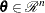can be subject to a set of m linear equality and inequality constraints: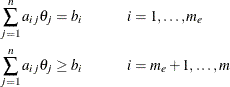The coefficients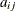and right-hand sides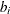of the equality and inequality constraints are collected in thematrix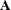and the m vector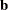.

The m linear constraints define a feasible region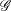in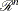that must contain the point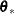that minimizes the problem. If the feasible regionis empty, no solution to the optimization problem exists.

In PROC NLMIXED, all optimization techniques use active set methods. The iteration starts with a feasible point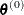, which you can provide or which can be computed by the Schittkowski and Stoer (1979) algorithm implemented in PROC NLMIXED. The algorithm then moves from one feasible point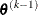to a better feasible point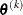along a feasible search direction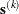,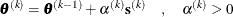Theoretically, the path of pointsnever leaves the feasible regionof the optimization problem, but it can reach its boundaries. The active set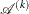of pointis defined as the index set of all linear equality constraints and those inequality constraints that are satisfied at. If no constraint is active, the point is located in the interior of, and the active setis empty. If the pointin iteration k hits the boundary of inequality constraint i, this constraint i becomes active and is added to. Each equality constraint and each active inequality constraint reduce the dimension (degrees of freedom) of the optimization problem.

In practice, the active constraints can be satisfied only with finite precision. The LCEPSILON=r option specifies the range for active and violated linear constraints. If the pointsatisfies the condition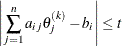where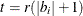, the constraint i is recognized as an active constraint. Otherwise, the constraint i is either an inactive inequality or a violated inequality or equality constraint. Due to rounding errors in computing the projected search direction, error can be accumulated so that an iteratesteps out of the feasible region.

In those cases, PROC NLMIXED might try to pull the iterateback into the feasible region. However, in some cases the algorithm needs to increase the feasible region by increasing the LCEPSILON=r value. If this happens, a message is displayed in the log output.

If the algorithm cannot improve the value of the objective function by moving from an active constraint back into the interior of the feasible region, it makes this inequality constraint an equality constraint in the next iteration. This means that the active set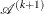still contains the constraint i. Otherwise, it releases the active inequality constraint and increases the dimension of the optimization problem in the next iteration.

A serious numerical problem can arise when some of the active constraints become (nearly) linearly dependent. PROC NLMIXED removes linearly dependent equality constraints before starting optimization. You can use the LCSINGULAR= option to specify a criterion r used in the update of the QR decomposition that determines whether an active constraint is linearly dependent relative to a set of other active constraints.

If the solution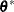is subjected to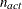linear equality or active inequality constraints, the QR decomposition of the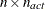matrix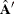of the linear constraints is computed by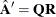, where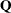is anorthogonal matrix and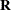is anupper triangular matrix. The n columns of matrixcan be separated into two matrices,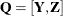, where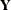contains the firstorthogonal columns ofandcontains the lastorthogonal columns of. The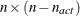column-orthogonal matrixis also called the null-space matrix of the active linear constraints. The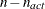columns of the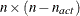matrixform a basis orthogonal to the rows of the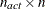matrix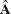.

At the end of the iterating, PROC NLMIXED computes the projected gradient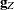,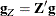In the case of boundary-constrained optimization, the elements of the projected gradient correspond to the gradient elements of the free parameters. A necessary condition forto be a local minimum of the optimization problem is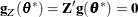The symmetric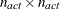matrix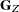,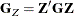is called a projected Hessian matrix. A second-order necessary condition forto be a local minimizer requires that the projected Hessian matrix is positive semidefinite.

Those elements of thevector of first-order estimates of Lagrange multipliers,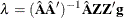that correspond to active inequality constraints indicate whether an improvement of the objective function can be obtained by releasing this active constraint. For minimization, a significant negative Lagrange multiplier indicates that a possible reduction of the objective function can be achieved by releasing this active linear constraint. The LCDEACT=r option specifies a threshold r for the Lagrange multiplier that determines whether an active inequality constraint remains active or can be deactivated. (In the case of boundary-constrained optimization, the Lagrange multipliers for active lower (upper) constraints are the negative (positive) gradient elements corresponding to the active parameters.)